# Enigmatic Perl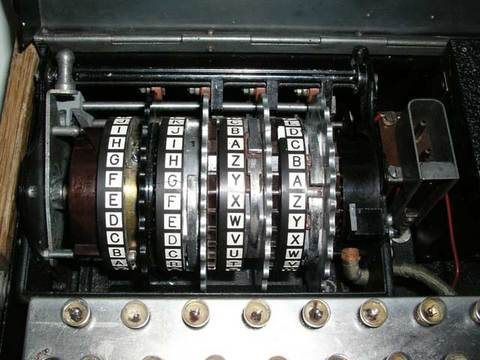• Enigmatic: of, relating to or resembling an enigma
• Enigma: something puzzling, perplexing, inexplicable
• Embrace them
• Eschew them
• Sit somewhere in the middle
• Rip them out

# \$|++• perlvar says:
• If set to nonzero, forces a flush right away and after every write or print on the currently selected output channel.
• \$| = 1;
• \$|++;
• Are these doing the same thing?
• zero or nonzero?

# Wraparound• Initial value: 0
• Multiple increments still nonzero
• Wraparound?
• use integer;
• Promotion to double
• Keep going until incremented value is indistinguishable

# Negative values• \$| = -1;
• \$|--;

# Let's experiment!• Assuming a 32 bit machine
• perl -le '\$|++ for 1 .. 2**32; print \$|'
• Range iterator outside integer range at -e line 1.
• Oops
• perl -le '\$|++ for 1 .. 2**31; print \$|'
• Range iterator outside integer range at -e line 1.

# Let's experiment!• Let's start simply
• perl -le '\$|++ for 1 .. 5; print \$|'
• 1
• WHAT?
• perl -le '\$x++ for 1 .. 5; print \$x'
• 5

# Let's experiment!• perl -le 'print \$|++ for 1 .. 5'
• 0
1
1
1
1
• Weird
• perl -le '\$| = 2; print \$|'
• 1

# Let's experiment!• perl -le '\$| = -1; print \$|'
• 1
• perl -le 'print \$|-- for 1 .. 5'
• 0
1
0
1
0

# Just a bit• \$|++ is functionally equivalent to \$| = 1
• Why?
• It's just a bit in a data structure masquerading as a variable

# Select a select• Need to select the correct filehandle for \$|
• Two flavours of select
• Select the first select
• Sets the current default filehandle for output and returns the previously selected filehandle
• This isn't completely accurate

# Select a filehandle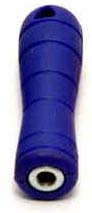my \$oldh = select(\$h);
\$| = 1;
select(\$oldh);

# Select a filehandle{
my \$oldh = select(\$h);
\$| = 1;
select(\$oldh);
}

# Select a filehandle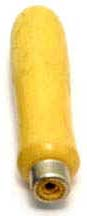{ my \$oldh = select(\$h); \$| = 1; select(\$oldh) }

# Select a filehandle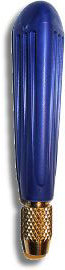select((select(\$h), \$| = 1));

# Break it up• select((select(\$h), \$| = 1));
• select((select(\$h), \$| = 1));
• select((select(\$h), \$| = 1));
• select((select(\$h), \$| = 1));
• select((select(\$h), \$| = 1));
• select((select(\$h), \$| = 1));

# Heavy, yet gracefuluse IO::Handle;
\$h->autoflush(1);

# Diamonds• while (<>) { }
• perl -MO=Deparse -e 'while (<>) {}'
• while (defined(\$_ = <ARGV>)) {
();
}
-e syntax OK
• <ARGV> is <>
• explicit assignment to \$_
• defined test
• new with perl-5.005

# List length• \$_ = "1234567890";
• how many times does /.../ match?

# Temporary arraymy @tmp = /.../g;
my \$count = @tmp;
• Gives 3 as expected

# No temporary arraymy \$count = /.../g;
• Oops - that gives 1
• Called in scalar context

# List contextmy (\$count) = /.../g;
• Oops - that gives 123
• Returns first match

# If all else fails, read the docs• List assignment in scalar context returns the number of elements produced by the expression on the right side of the assignment (perldata)
• while (my (\$key, \$val) = each %hash) { ... }
• We need list assignment in scalar context

# Scalar context• The scalar part is easy
• my \$count = ...

# Try againmy \$count = my @tmp = /.../g;
• Correct - that gives 3
• But we still have that ugly temporary array

# Assign to a listmy \$count = (my \$dummy) = /.../g;
• Correct - that gives 3
• But now we have an ugly temporary scalar

# Assign to undefmy \$count = (undef) = /.../g;
• Correct - that gives 3
• But it's still a bit ugly

# Assign to empty listmy \$count = () = /.../g;
• Correct - that gives 3
• And it doesn't look too bad

# Kill whitespacemy \$count =()= /.../g;
• Correct - that gives 3
• =()= pseudo-operator

# Line endings• \$/ - Input Record Separator
• To set it temporarily, use local
• Value is a string, "awk has to be better for something"
• Special values
• undef
• ""
• local \$/;
• local \$/ = \16;

# Little lists• for (\$val) { }
• What? Why?
• foreach and for
• \$_ aliases each list element
• braces show scope

# Example\$_ = 1;
my \$x = "xyz";
for (\$x)
{
s/x/a/;
s/y/--/;
s/z/length/e;
}
print "\$_:\$x";
• This prints out 1:a--4

# Command line• perl -pi.bak -e 's/Pb/Au/' *
• How do we do that within a program?
• system?

# Exampleperl -pi.bak -e 's/Pb/Au/' *

...
{
local \$^I = ".bak";
local @ARGV = glob "*";
while (<>)
{
s/Pb/Au/;
print;
}
}
...

# Or maybe something a little shorterperl -pi.bak -e 's/Pb/Au/' *

...
{
local (\$^I, @ARGV) = (".bak", glob "*");
s/Pb/Au/, print while <>;
}
...

# Select a second select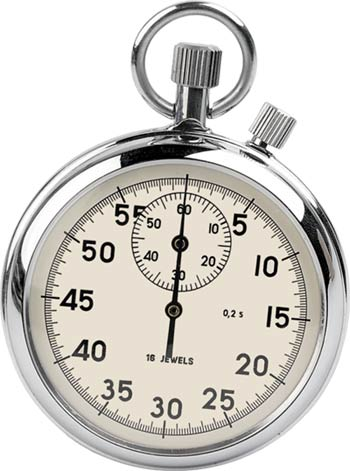• for (1 .. 1_000_000) { }
• sleep 3;
• select(2)
• select(undef, undef, undef, 0.5);

# Conclusion• There's all sorts of Perl code
• Some people can make Perl look like C
• or Java
• or Bourne shell
• or OCaml